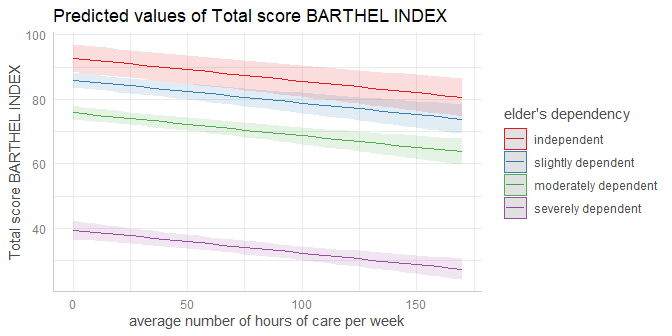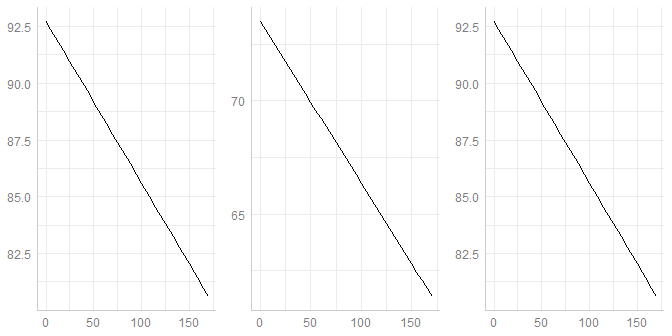# Technical Details: Difference between ggpredict() and ggemmeans()

#### 2021-03-17

ggpredict() and ggemmeans() compute predicted values for all possible levels or values from a model’s predictor. Basically, ggpredict() wraps the predict()-method for the related model, while ggemmeans() wraps the emmeans()-method from the emmeans-package. Both ggpredict() and ggemmeans() do some data-preparation to bring the data in shape for the newdata-argument (predict()) resp. the at-argument (emmeans()). It is recommended to read the general introduction first, if you haven’t done this yet.

Thus, effects returned by ggpredict() conditional effects (i.e. these are conditioned on certain (reference) levels of factors), while ggemmeans() returns marginal means, since the effects are “marginalized” (or “averaged”) over the levels of factors.

For models without categorical predictors, the results from ggpredict() and ggemmeans() are identical (except some slight differences in the associated confidence intervals, which are, however, negligible).

library(magrittr)
library(ggeffects)
data(efc)
fit <- lm(barthtot ~ c12hour + neg_c_7, data = efc)

ggpredict(fit, terms = "c12hour")
#> # Predicted values of Total score BARTHEL INDEX
#> # x = average number of hours of care per week
#>
#>   x | Predicted |         95% CI
#> --------------------------------
#>   0 |     75.07 | [72.96, 77.18]
#>  20 |     70.15 | [68.40, 71.91]
#>  45 |     64.01 | [62.41, 65.61]
#>  65 |     59.09 | [57.32, 60.86]
#>  85 |     54.17 | [52.04, 56.30]
#> 105 |     49.25 | [46.65, 51.86]
#> 125 |     44.34 | [41.18, 47.49]
#> 170 |     33.27 | [28.79, 37.76]
#>
#> * neg_c_7 = 11.83

ggemmeans(fit, terms = "c12hour")
#> # Predicted values of Total score BARTHEL INDEX
#> # x = average number of hours of care per week
#>
#>   x | Predicted |         95% CI
#> --------------------------------
#>   0 |     75.07 | [72.96, 77.19]
#>  20 |     70.15 | [68.40, 71.91]
#>  45 |     64.01 | [62.40, 65.61]
#>  65 |     59.09 | [57.32, 60.86]
#>  85 |     54.17 | [52.04, 56.31]
#> 105 |     49.25 | [46.64, 51.87]
#> 125 |     44.34 | [41.18, 47.49]
#> 170 |     33.27 | [28.78, 37.76]
#>
#> * neg_c_7 = 11.83

As can be seen, the continuous predictor neg_c_7 is held constant at its mean value, 11.83. For categorical predictors, ggpredict() and ggemmeans() behave differently. While ggpredict() uses the reference level of each categorical predictor to hold it constant, ggemmeans() - like ggeffect() - averages over the proportions of the categories of factors.

library(sjmisc)
data(efc)
efc$e42dep <- to_label(efc$e42dep)
fit <- lm(barthtot ~ c12hour + neg_c_7 + e42dep, data = efc)

ggpredict(fit, terms = "c12hour")
#> # Predicted values of Total score BARTHEL INDEX
#> # x = average number of hours of care per week
#>
#>   x | Predicted |         95% CI
#> --------------------------------
#>   0 |     92.74 | [88.48, 97.00]
#>  20 |     91.32 | [87.07, 95.57]
#>  45 |     89.53 | [85.21, 93.86]
#>  65 |     88.10 | [83.65, 92.56]
#>  85 |     86.68 | [82.04, 91.32]
#> 105 |     85.25 | [80.38, 90.12]
#> 125 |     83.82 | [78.67, 88.97]
#> 170 |     80.61 | [74.72, 86.50]
#>
#> * neg_c_7 =       11.83
#> *  e42dep = independent

ggemmeans(fit, terms = "c12hour")
#> # Predicted values of Total score BARTHEL INDEX
#> # x = average number of hours of care per week
#>
#>   x | Predicted |         95% CI
#> --------------------------------
#>   0 |     73.51 | [71.85, 75.18]
#>  20 |     72.09 | [70.65, 73.53]
#>  45 |     70.30 | [68.89, 71.71]
#>  65 |     68.87 | [67.29, 70.46]
#>  85 |     67.45 | [65.55, 69.34]
#> 105 |     66.02 | [63.74, 68.30]
#> 125 |     64.59 | [61.88, 67.31]
#> 170 |     61.38 | [57.61, 65.15]
#>
#> * neg_c_7 = 11.83

In this case, one would obtain the same results for ggpredict() and ggemmeans() again, if condition is used to define specific levels at which variables, in our case the factor e42dep, should be held constant.

ggpredict(fit, terms = "c12hour")
#> # Predicted values of Total score BARTHEL INDEX
#> # x = average number of hours of care per week
#>
#>   x | Predicted |         95% CI
#> --------------------------------
#>   0 |     92.74 | [88.48, 97.00]
#>  20 |     91.32 | [87.07, 95.57]
#>  45 |     89.53 | [85.21, 93.86]
#>  65 |     88.10 | [83.65, 92.56]
#>  85 |     86.68 | [82.04, 91.32]
#> 105 |     85.25 | [80.38, 90.12]
#> 125 |     83.82 | [78.67, 88.97]
#> 170 |     80.61 | [74.72, 86.50]
#>
#> * neg_c_7 =       11.83
#> *  e42dep = independent

ggemmeans(fit, terms = "c12hour", condition = c(e42dep = "independent"))
#> # Predicted values of Total score BARTHEL INDEX
#> # x = average number of hours of care per week
#>
#>   x | Predicted |         95% CI
#> --------------------------------
#>   0 |     92.74 | [88.48, 97.01]
#>  20 |     91.32 | [87.06, 95.57]
#>  45 |     89.53 | [85.20, 93.87]
#>  65 |     88.10 | [83.64, 92.57]
#>  85 |     86.68 | [82.03, 91.32]
#> 105 |     85.25 | [80.37, 90.13]
#> 125 |     83.82 | [78.67, 88.98]
#> 170 |     80.61 | [74.71, 86.51]
#>
#> * neg_c_7 = 11.83

Creating plots is as simple as described in the vignette Plotting Marginal Effects.

ggemmeans(fit, terms = c("c12hour", "e42dep")) %>% plot()But when should I use ggemmeans() and when ggpredict()?

When you are interested in the strength of association, it usually doesn’t matter. as you can see in the plots below. The slope of our focal term, c12hour, is the same for all three plots:

library(see)
p1 <- plot(ggpredict(fit, terms = "c12hour"), ci = FALSE, show.title = FALSE, show.x.title = FALSE, show.y.title = FALSE)
p2 <- plot(ggemmeans(fit, terms = "c12hour"), ci = FALSE, show.title = FALSE, show.x.title = FALSE, show.y.title = FALSE)
p3 <- plot(ggemmeans(fit, terms = "c12hour", condition = c(e42dep = "independent")), ci = FALSE, show.title = FALSE, show.x.title = FALSE, show.y.title = FALSE)

plots(p1, p2, p3, n_rows = 1)However, the predicted outcome varies. This gives an impression when ggemmeans(), i.e. marginal effects, matter: when you want to predict your outcome, marginalized over the different levels of factors, i.e. “generalized” to the population (of your sample). ggpredict() would give a predicted outcome for a subgroup of your sample, i.e. conditioned on specific levels of factors. Hence, the predicted outcome from ggpredict() does not necessarily generalize to the “population” (always keeping in mind that we assume having a “representative sample” of a “population” as data in our model).

But why should I use ggpredict() anymore?

Some models are not yet supported by the emmeans package, thus, for certain models, only ggpredict() works, not ggemmeans() nor ggeffect(). Sometimes, robust variance-covariance estimation is required for confidence intervals of predictions. In such cases, you have to rely on ggpredict(). If you have no categorical predictors as non-focal terms (i.e. no factor needs to be held constant), then - as shown above - ggpredict() and ggemmeans() yield the same results.